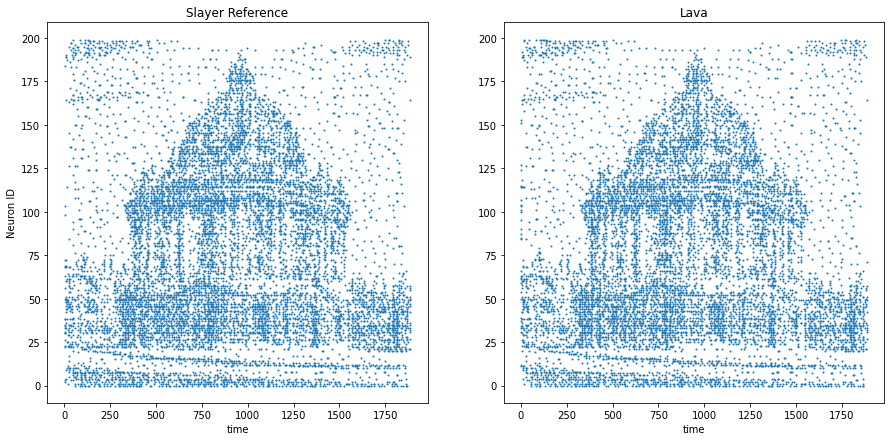# Oxford example

This tutorial demonstrates lava.lib.dl.netx api for running Oxford network trained using lava.lib.dl.slayer. The training example can be found here here

The task is to learn to transform a random Poisson spike train to produce output spike pattern that resembles The Radcliffe Camera building of Oxford University, England. The input and output both consist of 200 neurons each and the spikes span approximately 1900ms. The input and output pair are converted from SuperSpike (© GPL-3).

 Input Output:

import numpy as np
import matplotlib.pyplot as plt

from lava.magma.core.run_configs import Loihi1SimCfg
from lava.magma.core.run_conditions import RunSteps
from lava.proc.io.sink import RingBuffer as ReceiveProcess
from lava.proc.io.source import RingBuffer as SendProcess


## Network Exchange (NetX) Libarary

NetX api allows automatic creation of Lava process from the network specification. It is available as a part of Lava-DL library as lava.lib.dl.netx

:

from lava.lib.dl import netx
from lava.lib.dl import slayer


## Create network block

A Lava process describing the network can be created by simply instantiating netx.hdf5.Network with the path of the desired hdf5 network description file. * The input layer is accessible as net.in_layer. * The output layer is accessible as net.out_layer. * All the constituent layers are accessible as as a list: net.layers.

:

net = netx.hdf5.Network(net_config='Trained/network.net')
print(net)

|   Type   |  W  |  H  |  C  | ker | str | pad | dil | grp |delay|
|Dense     |    1|    1|  256|     |     |     |     |     |False|
|Dense     |    1|    1|  200|     |     |     |     |     |False|

:

print(f'There are {len(net)} layers in network:')

for l in net.layers:
print(f'{l.block:5s} : {l.name:10s}, shape : {l.shape}')

There are 2 layers in network:
Dense : Process_1 , shape : (256,)
Dense : Process_4 , shape : (200,)


## Create Spike Input/Ouptut process

Here, we will use RingBuffer process in lava.proc.io.{source/sink} to generate spike that is sent to the network and record the spike output from the network.

There are 200 neurons and the input spikes span apprximately 2000 steps.

We will use slayer.io utilities to read the event data and convert them to dense spike data.

:

input = slayer.io.read_np_spikes('input.npy')
source = SendProcess(input.to_tensor(dim=(1, 200, 2000)).squeeze())
source.s_out.connect(net.inp)
net.out.connect(sink.a_in)


## Run the network

We will run the network for 2000 steps and read the network’s output.

:

run_condition = RunSteps(num_steps=2000)
run_config = Loihi1SimCfg(select_tag='fixed_pt')
net.run(condition=run_condition, run_cfg=run_config)
output = sink.data.get()
net.stop()


## Plot the results

First, convert output spike data into an event and plot them

:

out_event = slayer.io.tensor_to_event(output.reshape((1,) + output.shape))

:

fig, ax = plt.subplots(1, 2, figsize=(15, 7))
ax.plot(gt_event.t, gt_event.x, '.', markersize=2)
ax.plot(out_event.t, out_event.x, '.', markersize=2)
ax.set_title('Slayer Reference')
ax.set_ylabel('Neuron ID')
ax.set_xlabel('time')
ax.set_title('Lava')
ax.set_xlabel('time')

:

Text(0.5, 0, 'time')